教程：使用示例 Jupyter Notebook 训练图像分类模型

• 设置开发环境。
• 访问和检查数据。
• 在远程群集上训练一个简单的逻辑回归模型。
• 查看定型结果，然后注册最佳模型。

先决条件

• 完成快速入门：Azure 机器学习入门以执行以下操作：
• 创建工作区。
• 创建用于开发环境的基于云的计算实例。
• 创建用于训练模型的基于云的计算群集。

在工作区中运行笔记本

Azure 机器学习在你的工作区中提供了一个云笔记本服务器，实现了免安装的预配置体验。 如果你希望控制环境、包和依赖项，请使用自己的环境

克隆笔记本文件夹

1. 登录到 Azure 机器学习工作室

2. 选择创建的订阅和工作区。

3. 在左侧选择“笔记本”。

4. 在顶部选择“示例”选项卡。

5. 打开“Python”文件夹。

6. 打开包含版本号的文件夹。 此数字表示 Python SDK 的当前版本。

7. 选择“教程”文件夹右侧的“…”按钮，然后选择“克隆” 。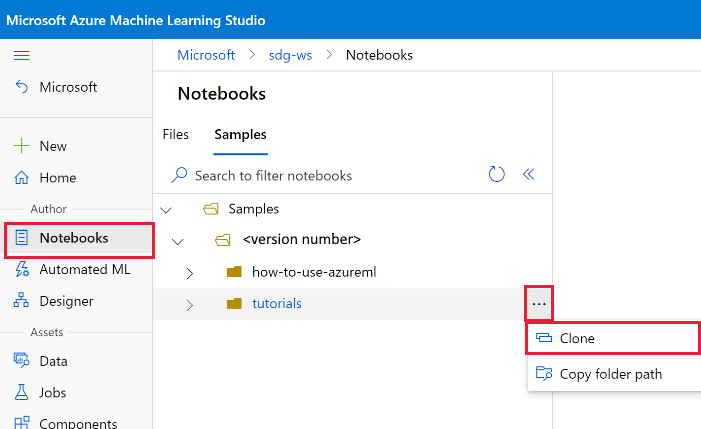8. 文件夹列表显示访问该工作区的每个用户。 选择要将“tutorials”文件夹克隆到其中的文件夹。

打开克隆的笔记本

1. 打开已克隆到“用户文件”部分的“tutorials”文件夹 。

重要

可以查看“示例”文件夹中的笔记本，但无法从此文件夹运行笔记本。 若要运行笔记本，请确保在“用户文件”部分打开笔记本的克隆版本。

2. 选择 tutorials/image-classification-mnist-data 文件夹中的 img-classification-part1-training.ipynb 文件。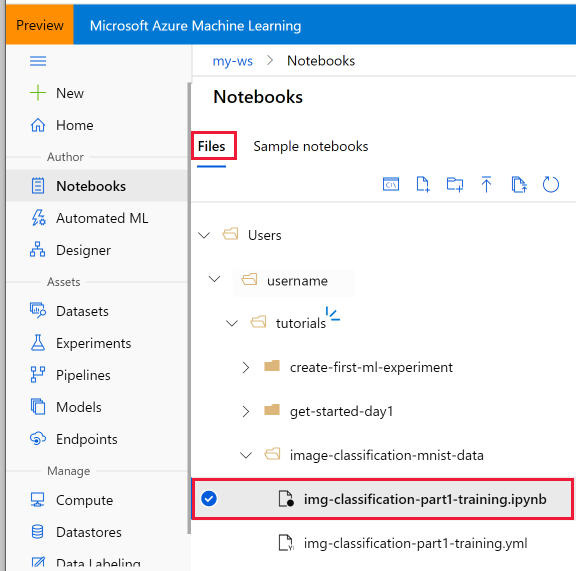3. 在顶部栏上，选择用于运行笔记本的计算实例。

设置开发环境

• 导入 Python 包。
• 连接到工作区，以便本地计算机能够与远程资源通信。
• 创建试验来跟踪所有运行。
• 创建要用于定型的远程计算目标。

导入程序包

%matplotlib inline
import numpy as np
import matplotlib.pyplot as plt

import azureml.core
from azureml.core import Workspace

# check core SDK version number
print("Azure ML SDK Version: ", azureml.core.VERSION)

连接到工作区

# load workspace configuration from the config.json file in the current folder.
ws = Workspace.from_config()
print(ws.name, ws.location, ws.resource_group, sep='\t')

创建试验

from azureml.core import Experiment
experiment_name = 'Tutorial-sklearn-mnist'

exp = Experiment(workspace=ws, name=experiment_name)

创建或附加现有的计算目标

Azure 机器学习计算是一项托管服务，可让数据科学家在 Azure 虚拟机群集上训练机器学习模型。 示例包括带 GPU 支持的 VM。 在本教程中，你将创建 Azure 机器学习计算作为训练环境。 在本教程的后面部分，你将提交要在此 VM 上运行的 Python 代码。

from azureml.core.compute import AmlCompute
from azureml.core.compute import ComputeTarget
import os

# choose a name for your cluster
compute_name = os.environ.get("AML_COMPUTE_CLUSTER_NAME", "cpu-cluster")
compute_min_nodes = os.environ.get("AML_COMPUTE_CLUSTER_MIN_NODES", 0)
compute_max_nodes = os.environ.get("AML_COMPUTE_CLUSTER_MAX_NODES", 4)

# This example uses CPU VM. For using GPU VM, set SKU to STANDARD_NC6
vm_size = os.environ.get("AML_COMPUTE_CLUSTER_SKU", "STANDARD_D2_V2")

if compute_name in ws.compute_targets:
compute_target = ws.compute_targets[compute_name]
if compute_target and type(compute_target) is AmlCompute:
print('found compute target. just use it. ' + compute_name)
else:
print('creating a new compute target...')
provisioning_config = AmlCompute.provisioning_configuration(vm_size=vm_size,
min_nodes=compute_min_nodes,
max_nodes=compute_max_nodes)

# create the cluster
compute_target = ComputeTarget.create(
ws, compute_name, provisioning_config)

# can poll for a minimum number of nodes and for a specific timeout.
# if no min node count is provided it will use the scale settings for the cluster
compute_target.wait_for_completion(
show_output=True, min_node_count=None, timeout_in_minutes=20)

# For a more detailed view of current AmlCompute status, use get_status()
print(compute_target.get_status().serialize())

浏览数据

• 下载 MNIST 数据集。
• 显示一些示例图像。

下载 MNIST 数据集

from azureml.core import Dataset
from azureml.opendatasets import MNIST

data_folder = os.path.join(os.getcwd(), 'data')
os.makedirs(data_folder, exist_ok=True)

mnist_file_dataset = MNIST.get_file_dataset()

mnist_file_dataset = mnist_file_dataset.register(workspace=ws,
name='mnist_opendataset',
description='training and test dataset',
create_new_version=True)

显示一些示例图像

# make sure utils.py is in the same directory as this code
import glob

# note we also shrink the intensity values (X) from 0-255 to 0-1. This helps the model converge faster.
X_train = load_data(glob.glob(os.path.join(data_folder,"**/train-images-idx3-ubyte.gz"), recursive=True), False) / 255.0
X_test = load_data(glob.glob(os.path.join(data_folder,"**/t10k-images-idx3-ubyte.gz"), recursive=True), False) / 255.0

# now let's show some randomly chosen images from the traininng set.
count = 0
sample_size = 30
plt.figure(figsize=(16, 6))
for i in np.random.permutation(X_train.shape)[:sample_size]:
count = count + 1
plt.subplot(1, sample_size, count)
plt.axhline('')
plt.axvline('')
plt.text(x=10, y=-10, s=y_train[i], fontsize=18)
plt.imshow(X_train[i].reshape(28, 28), cmap=plt.cm.Greys)
plt.show()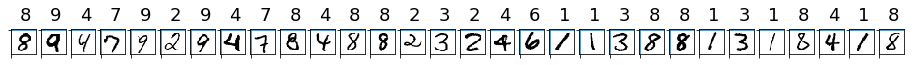在远程群集上定型

• 创建目录
• 创建定型脚本
• 创建脚本运行配置
• 提交作业

创建目录

import os
script_folder = os.path.join(os.getcwd(), "sklearn-mnist")
os.makedirs(script_folder, exist_ok=True)

创建定型脚本

%%writefile \$script_folder/train.py

import argparse
import os
import numpy as np
import glob

from sklearn.linear_model import LogisticRegression
import joblib

from azureml.core import Run

# let user feed in 2 parameters, the dataset to mount or download, and the regularization rate of the logistic regression model
parser = argparse.ArgumentParser()
parser.add_argument('--data-folder', type=str, dest='data_folder', help='data folder mounting point')
parser.add_argument('--regularization', type=float, dest='reg', default=0.01, help='regularization rate')
args = parser.parse_args()

data_folder = args.data_folder
print('Data folder:', data_folder)

# load train and test set into numpy arrays
# note we scale the pixel intensity values to 0-1 (by dividing it with 255.0) so the model can converge faster.
X_train = load_data(glob.glob(os.path.join(data_folder, '**/train-images-idx3-ubyte.gz'), recursive=True), False) / 255.0
X_test = load_data(glob.glob(os.path.join(data_folder, '**/t10k-images-idx3-ubyte.gz'), recursive=True), False) / 255.0
y_train = load_data(glob.glob(os.path.join(data_folder, '**/train-labels-idx1-ubyte.gz'), recursive=True), True).reshape(-1)
y_test = load_data(glob.glob(os.path.join(data_folder, '**/t10k-labels-idx1-ubyte.gz'), recursive=True), True).reshape(-1)
print(X_train.shape, y_train.shape, X_test.shape, y_test.shape, sep = '\n')

# get hold of the current run
run = Run.get_context()

print('Train a logistic regression model with regularization rate of', args.reg)
clf = LogisticRegression(C=1.0/args.reg, solver="liblinear", multi_class="auto", random_state=42)
clf.fit(X_train, y_train)

print('Predict the test set')
y_hat = clf.predict(X_test)

# calculate accuracy on the prediction
acc = np.average(y_hat == y_test)
print('Accuracy is', acc)

run.log('regularization rate', np.float(args.reg))
run.log('accuracy', np.float(acc))

os.makedirs('outputs', exist_ok=True)
# note file saved in the outputs folder is automatically uploaded into experiment record
joblib.dump(value=clf, filename='outputs/sklearn_mnist_model.pkl')

• 定型脚本读取参数以查找包含数据的目录。 稍后提交作业时，请参考数据存储获取此参数：parser.add_argument('--data-folder', type=str, dest='data_folder', help='data directory mounting point')

• 训练脚本将模型保存到一个名为 outputs 的目录中。 此目录中编写的所有内容都会自动上传到你的工作区。 稍后，你将在本教程从此目录访问模型。 joblib.dump(value=clf, filename='outputs/sklearn_mnist_model.pkl')

• 训练脚本需要使用文件 utils.py 来正确加载数据集。 以下代码将 utils.py 复制到 script_folder 中，以便可以与远程资源上的训练脚本一起访问该文件。

import shutil
shutil.copy('utils.py', script_folder)

配置训练作业

• 包含脚本的目录。 此目录中的所有文件都上传到群集节点以便执行。
• 计算目标。 在此示例中，将使用所创建的 Azure 机器学习计算群集。
• 定型脚本名称，train.py。
• 一个环境，其中包含运行脚本所需的库。
• 训练脚本中的必需参数。

from azureml.core.environment import Environment

# to install required packages
env = Environment('tutorial-env')

env.python.conda_dependencies = cd

# Register environment to re-use later
env.register(workspace=ws)

from azureml.core import ScriptRunConfig

args = ['--data-folder', mnist_file_dataset.as_mount(), '--regularization', 0.5]

src = ScriptRunConfig(source_directory=script_folder,
script='train.py',
arguments=args,
compute_target=compute_target,
environment=env)

将作业提交到群集

run = exp.submit(config=src)
run

监视远程运行

• 映像创建：将创建与 Azure ML 环境指定的 Python 环境匹配的 Docker 映像。 映像将上传到工作区。 创建和上传映像需要大约五分钟。

此阶段针对每个 Python 环境发生一次，因为已缓存容器用于后续运行。 映像创建期间，日志将流式传输到运行历史记录。 可以使用这些日志监视映像创建进度。

• 缩放：如果远程群集需要比当前可用节点更多的节点来执行运行，则会自动添加其他节点。 缩放通常需要大约五分钟。

• 正在运行：在此阶段，必要的脚本和文件会发送到计算目标。 接着，装载或复制数据存储。 然后，运行 entry_script。 运行作业时，stdout 和 ./logs 目录会流式传输到运行历史记录 。 可以使用这些日志监视运行进度。

• 后期处理：运行的 ./outputs 目录将复制到工作区中的运行历史记录，以便可以访问这些结果。

Jupyter 小组件

from azureml.widgets import RunDetails
RunDetails(run).show()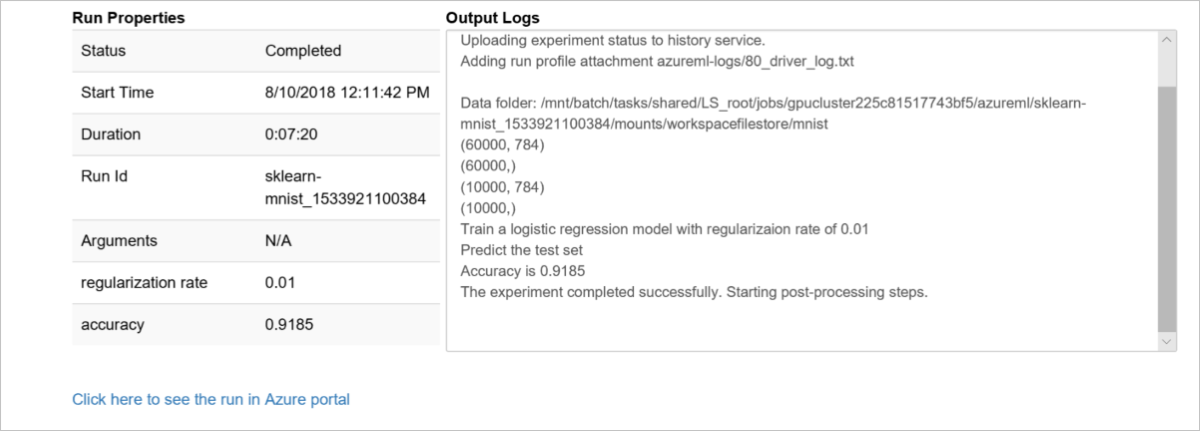完成时获取日志结果

run.wait_for_completion(show_output=False)  # specify True for a verbose log

显示运行结果

print(run.get_metrics())

{'regularization rate': 0.8, 'accuracy': 0.9204}

注册模型

print(run.get_file_names())

# register model
model = run.register_model(model_name='sklearn_mnist',
model_path='outputs/sklearn_mnist_model.pkl')
print(model.name, model.id, model.version, sep='\t')

清理资源

1. 在 Azure 门户中，选择最左侧的“资源组” 。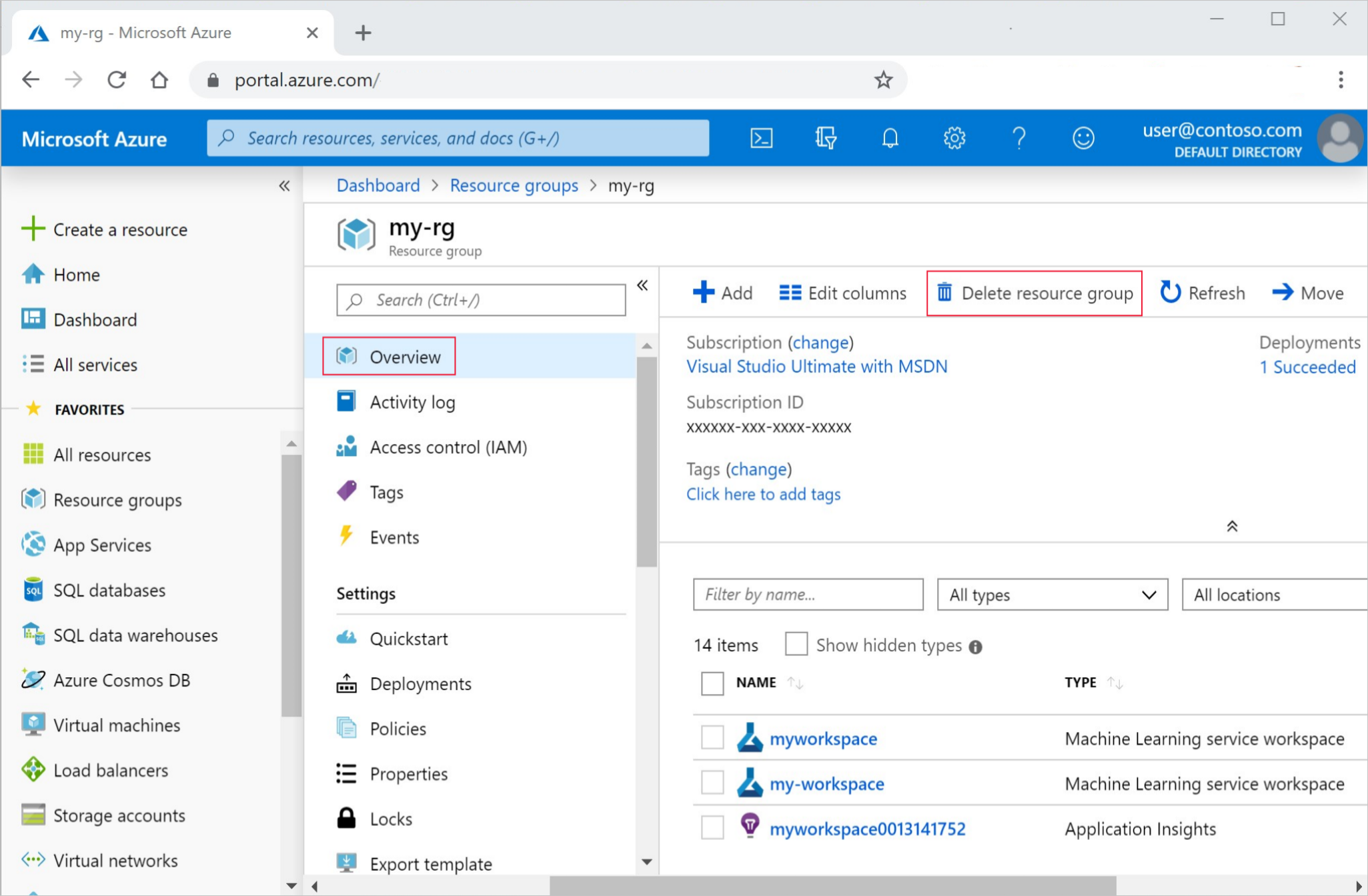2. 从列表中选择已创建的资源组。

3. 选择“删除资源组” 。

4. 输入资源组名称。 然后选择“删除” 。

# Optionally, delete the Azure Machine Learning Compute cluster
compute_target.delete()

后续步骤

• 设置开发环境。
• 访问和检查数据。
• 使用流行的 scikit-learn 机器学习库训练远程群集上的多个模型
• 查看定型详细信息，然后注册最佳模型。